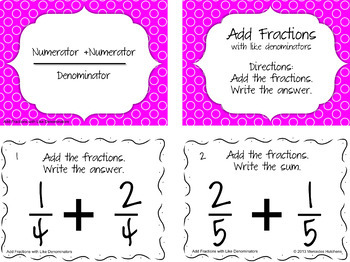# Add and Subtract Fractions and Simplify Task Card & PowerPoint BundleSubject
Resource Type
Common Core State Standards
Product Rating
File Type

Compressed Zip File

Be sure that you have an application to open this file type before downloading and/or purchasing.

20 MB|100 pages
Share
5 Products in this Bundle
5 products
1. Add Fractions with Like Denominators Task Cards Add Fractions with Like Denominators 4.NF.3 Objective: I will be able to add fractions with the same denominator These task cards can used as an early finisher, as a scoot game, or as a center. Included: 30 task cards Instruction and Hint task
2. Add Fractions with Like Denominators Then Simplify Task Cards Add Fractions with Like Denominators Then Simplify 4.NF.3 Objectives: I will be able to add fractions with the same denominator. I will be able to simplify fractions. These task cards can used as an early finisher, as a scoot game
3. Subtract Fractions with Like Denominators Task Cards Subtract Fractions with Like Denominators 4.NF.3 Objectives: I will be able to subtract fractions with the same denominator These task cards can used as an early finisher, as a scoot game, or as a center. Included: 30 task cards Instruct
4. Subtract Fractions with Like Denominators Then Simplify Task Cards Subtract Fractions with Like Denominators Then Simplify 4.NF.3 Objectives: I will be able to subtract fractions with the same denominator. I will be able to simplify fractions. These task cards can used as an early finisher, a
5. This PowerPoint can be used to introduce three strategies for simplifying fractions: using prime factors, using greatest common factors, and using mental division. This reviews vocabulary and provides foldables. The first 11 slides review vocabulary such as prime factors, greatest common factors, e
Bundle Description
Are your students starting to add fraction and subtract fractions with like denominators?This Add and Subtract Fractions and Simplify Bundle will give your students practice adding fractions with like denominators with and without simplifying (reducing fractions).

Save 20% by buying this bundle!

Add Fractions with Like Denominators 4.NF.3
Students will add fractions with the same denominator.

Add Fractions with Like Denominators Then Simplify 4.NF.3
Students will add fractions and simplify the answer.

Subtract Fractions with Like Denominators 4.NF.3
Students will subtract fractions.

Subtract Fractions with Like Denominators 4.NF.3
Students will subtract fractions. Students will simplify fractions.

Simplify Fractions PowerPoint 3.NF.3, 4.NF.3, 5.NF.1
Students will simplify fractions using prime factorization and common factors.

These task cards can used as an early finisher, as a scoot game, or as a center.

Included for each set of Task Cards:
Instruction and Hint task cards
Two recording sheets
Vocabulary Posters (can be shown on interactive white board or printed)
Objective Poster
Organizational Tips
A recording sheet to track student progress

The PowerPoint includes vocabulary, instructions, a foldable activity and student practice slides.

Check out my Equivalent Fraction Bundle which contains:
Identify Equivalent Fractions Using Models
Equivalent to One
Replace Mixed Numbers with an Improper Fraction
Replace Improper Fractions with a Mixed Number
and a game:
Equivalent Fractions Game and Vocabulary
Total Pages
100 pages
Included
Teaching Duration
N/A
Report this Resource
Reported resources will be reviewed by our team. Report this resource to let us know if this resource violates TpT’s content guidelines.